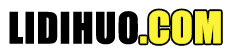# Statistics 调整后的 R 方

R 平方测量线性回归模型中由自变量 (X) 解释的因变量 (Y) 的变异比例。调整后的 R 平方根据模型中自变量的数量调整统计量。${R^2}$ 显示项（数据点）拟合曲线或直线的程度。调整后的 ${R^2}$ 还表示项与曲线或直线的拟合程度，但会根据模型中的项数进行调整。如果向模型中添加越来越多的无用变量，调整后的 r 平方将减小。如果添加更多有用的变量，调整后的 r 平方会增加。

## 公式

${R_{adj}^2 = 1-[\frac{(1-R^2)(n-1)}{n-k-1}]}$

${n}$ = 数据样本中的点数。 ${k}$ = 独立回归量的数量，即模型中变量的数量，不包括常量。

## 示例

${R_{adj}^2 = 1-[\frac{(1-0.5^2)(50-1)}{50-5-1}] \\[7pt] \, = 1-(0.75) \次 \frac{49}{44} , \\[7pt] \, = 1-0.8352 , \\[7pt] \, = 0.1648 }$